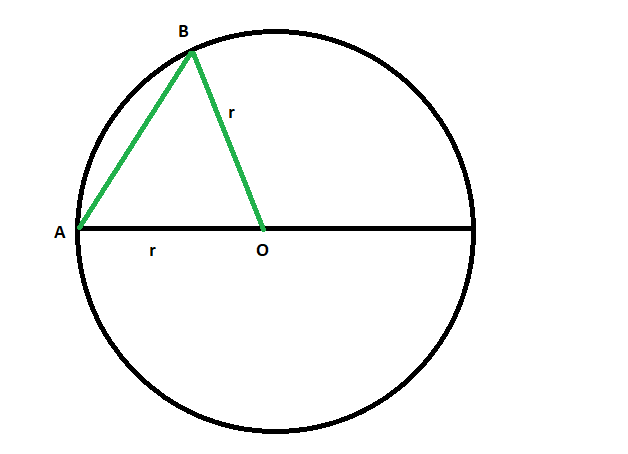# Find the Diameter or Longest chord of a Circle

Given a circle with radius ‘r’ is given, the task is to find the diameter or longest chord of the circle.

Examples:

```Input: r = 4
Output: 8

Input: r = 9
Output: 18
```Proof that the Longest chord of a circle is its Diameter:

• Draw circle O and any chord AB on it.
• From one endpoint of the chord, say A, draw a line segment through the centre. That is, draw a diameter.
• Now draw a radius from centre O to B.
• By the triangle inequality,
```AB < AO + OB
= r + r
= 2r
= d
```
• So, any chord that is not a diameter will be smaller than a diameter.
• So the largest chord is a diameter

Approach:

• The Longest chord of any circle is its diameter.
• Therefore, the diameter of a circle is twice the radius of it.
`Length of the longest chord or diameter = 2r`

Below is the implementation of the above approach:

## C++

 `// C++ program to find ` `// the longest chord or diameter ` `// of the circle whose radius is given ` ` `  `#include ` `using` `namespace` `std; ` ` `  `// Function to find the longest chord ` `void` `diameter(``double` `r) ` `{ ` `    ``cout << ``"The length of the longest chord"` `         ``<< ``" or diameter of the circle is "` `         ``<< 2 * r << endl; ` `} ` ` `  `// Driver code ` `int` `main() ` `{ ` ` `  `    ``// Get the radius ` `    ``double` `r = 4; ` ` `  `    ``// Find the diameter ` `    ``diameter(r); ` ` `  `    ``return` `0; ` `} `

## Java

 `// Java program to find ` `// the longest chord or diameter ` `// of the circle whose radius is given ` `class` `GFG  ` `{ ` `     `  `// Function to find the longest chord ` `static` `void` `diameter(``double` `r) ` `{ ` `    ``System.out.println(``"The length of the longest chord"` `        ``+ ``" or diameter of the circle is "` `        ``+ ``2` `* r); ` `} ` ` `  `// Driver code ` `public` `static` `void` `main(String[] args) ` `{ ` `     `  `    ``// Get the radius ` `    ``double` `r = ``4``; ` ` `  `    ``// Find the diameter ` `    ``diameter(r); ` `} ` `} ` ` `  `// This code contributed by Rajput-Ji `

## Python3

 `# Python3 program to find ` `# the longest chord or diameter ` `# of the circle whose radius is given ` ` `  `# Function to find the longest chord ` `def` `diameter(r): ` ` `  `    ``print``(``"The length of the longest chord"` `        ``,``" or diameter of the circle is "` `        ``,``2` `*` `r) ` ` `  ` `  `# Driver code ` ` `  `# Get the radius ` `r ``=` `4` ` `  `# Find the diameter ` `diameter(r) ` ` `  `# This code is contributed by mohit kumar `

## C#

 `// C# program to find ` `// the longest chord or diameter ` `// of the circle whose radius is given ` `using` `System; ` ` `  `class` `GFG  ` `{ ` `     `  `// Function to find the longest chord ` `static` `void` `diameter(``double` `r) ` `{ ` `    ``Console.WriteLine(``"The length of the longest chord"` `        ``+ ``" or diameter of the circle is "` `        ``+ 2 * r); ` `} ` ` `  `// Driver code ` `public` `static` `void` `Main(String[] args) ` `{ ` `     `  `    ``// Get the radius ` `    ``double` `r = 4; ` ` `  `    ``// Find the diameter ` `    ``diameter(r); ` `} ` `} ` ` `  `// This code has been contributed by 29AjayKumar `

## PHP

 ` `

Output:

```The length of the longest chord or diameter of the circle is 8
```

My Personal Notes arrow_drop_upBudding Web DeveloperKeen learnerAverage CoderDancer&Social Activist

If you like GeeksforGeeks and would like to contribute, you can also write an article using contribute.geeksforgeeks.org or mail your article to contribute@geeksforgeeks.org. See your article appearing on the GeeksforGeeks main page and help other Geeks.

Please Improve this article if you find anything incorrect by clicking on the "Improve Article" button below.

Article Tags :
Practice Tags :

Be the First to upvote.

Please write to us at contribute@geeksforgeeks.org to report any issue with the above content.